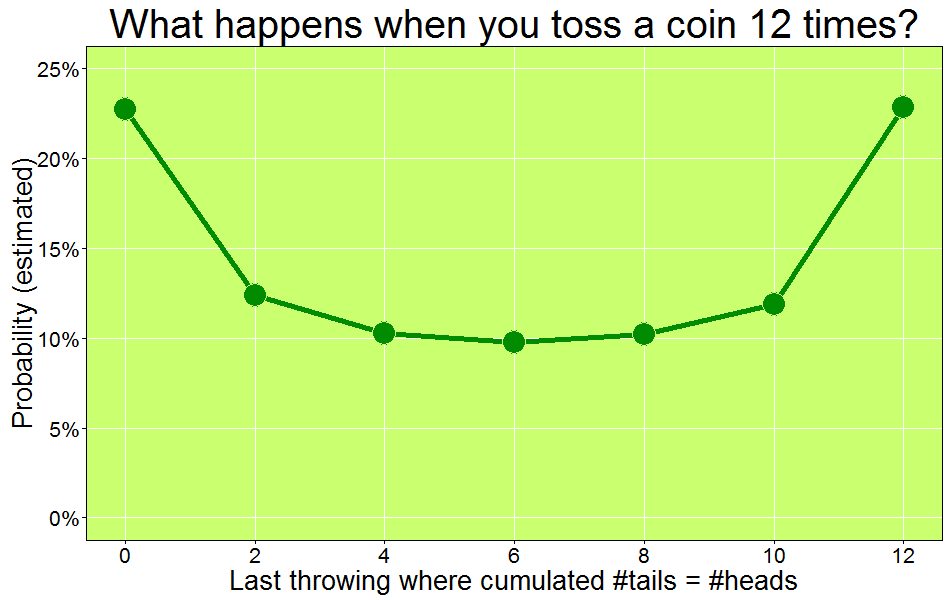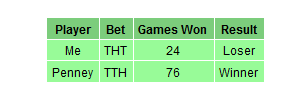# Amazing Things That Happen When You Toss a Coin 12 Times

If there is a God, he’s a great mathematician (Paul Dirac)

Imagine you toss a coin 12 times and you count how many heads and tails you are obtaining after each throwing (the coin is equilibrated so the probability of head or tail is the same). At some point, it can happen that number of heads and number of tails are the same. For example, if you obtain the sequence T-H-T-T-H-T-H-H-T-T-H-H, after the second throwing, number of heads is equal to number of tails (and both equal to one). It happens again after the 8th throwing and after last one. In this example, the last throwing where equallity occurs is the number 12. Obviously, equallity can only be observed in even throwings.

If you repeat the experiment 10.000 times you will find something like this if you draw the relative frequency of the last throwing where cumulated number of heads is equal to the one of tails:From my point of view there are three amazing things in this plot:

1. It is symmetrical, so `prob(n)=prob(12-n)`
2. The least likely throwing to obtain the last equality is the central one.
3. As a corollary, the most likely is not obtaining any equality (number of heads never are the same than number of tails) or obtaining last equality in the last throwing: two extremely different scenarios with the same chances to be observed.

Behind the simplicity of tossing coins there is a beautiful universe of mathematical surprises.

```library(dplyr)
library(ggplot2)
library(scales)
tosses=12
iter=10000
results=data.frame(nmax=numeric(0), count=numeric(0), iter=numeric(0))
tmp=data.frame(nmax=numeric(0))
for (j in 1:iter)
{
data.frame(x=sample(c(-1,1), size=tosses, replace=TRUE)) %>%
mutate(cumsum = cumsum(x)) %>% filter(cumsum==0) %>%
summarize(nmax=max(as.numeric(n))) %>% rbind(tmp)->tmp
}
tmp %>%
group_by(nmax) %>%
summarize(count=n()) %>%
mutate(nmax=ifelse(is.finite(nmax), nmax, 0), iter=iter) %>%
rbind(results)->results
opts=theme(
panel.background = element_rect(fill="darkolivegreen1"),
panel.border = element_rect(colour="black", fill=NA),
axis.line = element_line(size = 0.5, colour = "black"),
axis.ticks = element_line(colour="black"),
panel.grid.major = element_line(colour="white", linetype = 1),
panel.grid.minor = element_blank(),
axis.text.y = element_text(colour="black"),
axis.text.x = element_text(colour="black"),
text = element_text(size=20),
legend.key = element_blank(),
plot.title = element_text(size = 30)
)
ggplot(results, aes(x=nmax, y=count/iter)) +
geom_line(size=2, color="green4")+
geom_point(size=8, fill="green4", colour="darkolivegreen1",pch=21)+
scale_x_continuous(breaks = seq(0, tosses, by=2))+
scale_y_continuous(labels=percent, limits=c(0, .25))+
labs(title="What happens when you toss a coin 12 times?",
x="Last throwing where cumulated #tails = #heads",
y="Probability (estimated)")+opts
```

# Do Not Play With Mr. Penney

Facts do not speak (Henry Poincare)

Mr. Penney is my best friend. He is maths teacher and loves playing. Yesterday we were in his office at the university when he suggested me a game:

When you toss a coin three times, you can obtain eight different sequences of tails and heads: TTT, TTH, THT, HTT, THH, HTH, HHT and HHH. Using a fair coin, all sequences have the same chances to appear. Choose one sequence and I will then choose another one. I will toss a coin until either your or my sequence appears as a consecutive subsequence of the coin toss outcomes. The player whose sequence appears first wins. I will repeat this procedure 100 times. The one with more games won is the winner of the game.  Don’t worry: I will not toss the coin manually. I will simulate using my computer. What’s your bet?

Ok, my bet is THT, I said. After some seconds, Mr. Penney said: My bet is TTH.

This was the result of the first round:Another chance? told me Mr. Penney. Of course! Now my bet is TTH! I said. In fact, I was thinking Take that! Now I chose your previous bet. Do you think I am foolish?. After some seconds, Mr. Penney said: My bet now is HTT.

This was the result of the second round:Another chance? told me Mr. Penney. At this point, I was very suspicious but I wanted the last chance so I told him Of course! Now my bet is HTT! I wanted to try my strategy one more time. After some seconds, Mr. Penney said: My bet now is HHT.

This was the result of the third round:Ok, I give it up! What’s the trick? I said. And Mr. Penney explained it to me. You can find the explanation here. This is the last time I play with you! I told him once he finished the explanation.

Here you have the code. Feel free to play:

```library(gridExtra)
library(gridExtra)
Me     <- "TTH"
Penney <- "HTT"
results <- data.frame(play= numeric(0), Penney = integer(0), Me = character(0))
for (i in 1:100) {
play <- c()
repeat {play <- do.call(paste, c(play, as.list(sample(c("H","T"), 1)), sep=""))
if (grepl(Penney, play)|grepl(Me, play)) {
results <- rbind(results, data.frame(play= i, Penney = as.numeric(grepl(Penney, play)), Me = as.numeric(grepl(Me, play))))
break}}}
grid.newpage()
table <- rbind(
c("Me", Me, sum(results\$Me), if(sum(results\$Penney) > sum(results\$Me)) "Loser" else "Winner"),
c("Penney", Penney, sum(results\$Penney), if(sum(results\$Penney) > sum(results\$Me)) "Winner" else "Loser"))
grid.table(table,
cols = c("Player", "Bet", "Games Won", "Result"),
gpar.colfill = gpar(fill="palegreen3",col="White"),
gpar.corefill =  gpar(fill="palegreen",col="White"),
gpar.rowfill = gpar(fill=NA, col=NA))
```